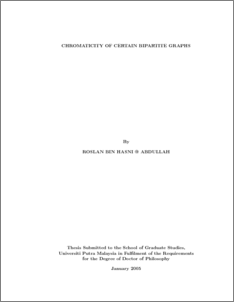# Chromaticity of Certain Bipartite Graphs

## Citation

Hasni @ Abdullah, Roslan (2005) Chromaticity of Certain Bipartite Graphs. PhD thesis, Universiti Putra Malaysia.

## Abstract

Since the introduction of the concepts of chromatically unique graphs and chromatically equivalent graphs, numerous families of such graphs have been obtained. The purpose of this thesis is to continue with the search of families of chromatically unique bipartite graphs. In Chapters 1 and 2, we define the concept of graph colouring, the associated chromatic polynomial and some properties of a chromatic polynomial. We also give some necessary conditions for graphs that are chromatically unique or chromatically equivalent. We end this chapter by stating some known results on the chromaticity of bipartite graphs, denoted as K(p,q).Preview
PDF
1000548936_fsas_2005_2.pdfView Item